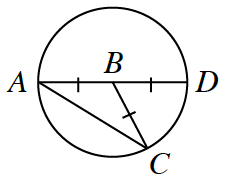### Home > CCG > Chapter 10 > Lesson 10.1.1 > Problem10-7

10-7.

In the diagram below, $\overline{AD}$ is a diameter of $⊙B$.1. If $m∠A = 35°$, what is $m∠CBD$?

$∠A$ is the inscribed angle and $∠CBD$ is the central angle intercepting the same arc.
How are these angles related?

$70°$

2. If $m∠CBD = 100°$, what is $m∠A$?

$50°$

3. If $m∠A = x$, what is $m∠CBD$?

Use the same method you used to solve part (a).